# A long wire carrying 4.50 A of current makes two 90 bends, as shown in the...

A long wire carrying 4.50 A of current makes two 90° bends, as shown in the figure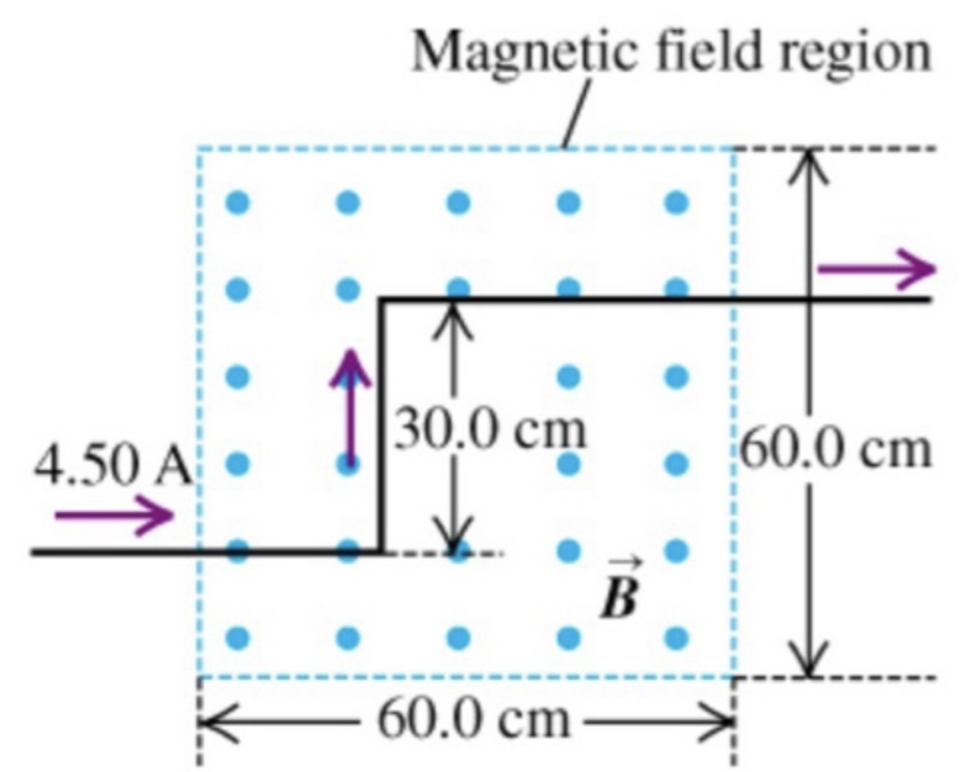The bent part of the wire passes through a uniform 0.238 T magnetic field directed as shown in the figure and confined to a limited region of space.

Part A

Find the magnitude of the force that the magnetic field experts on the the wire.

Part B

Find the direction of the force that the magnetic field experts on the the wire.

The magnetic force on a current carrying conductor $$F=B I L \sin \theta$$

For the horizontal region,

$$F_{H}=0.238 \times 4.5 \times 0.6=0.64 \mathrm{~N}$$

Direction: The magnetic field faces outside the plane of paper, current to the right, hence the force downwards.

For the vertical region,

$$F_{V}=0.238 \times 4.5 \times 0.3=0.32 \mathrm{~N}$$

Direction: The magnetic field faces outside the plane of paper, current to the top, hence the force right.

Total force, $$F=\sqrt{(0.64)^{2}+(0.32)^{2}}=0.72 \mathrm{~N}$$

Direction: Clockwise from the right $$45^{\circ}$$

The final direction angle is wrong...

You take the inverse tan of Fx/Fy

#### Earn Coin

Coins can be redeemed for fabulous gifts.

Similar Homework Help Questions
• ### A long wire carrying 4.50 A of current makes two 90 degree bends, as shown in....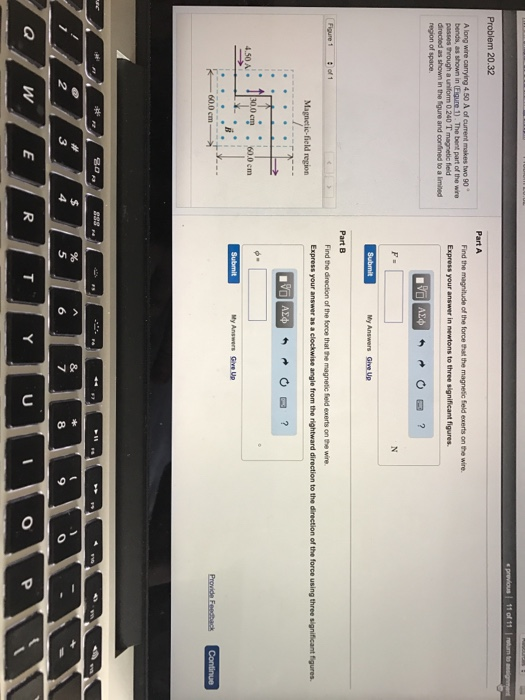A long wire carrying 4.50 A of current makes two 90 degree bends, as shown in. The best part of the wire passes through a uniform 0.240 t magnetic field directed as shown in the figure and confined to a limited of space. Part A Find the magnitude of the force that the magnetic field exerts on the wire Part B Find the direction of the force that the magnetic field exerts on the wire.

• ### Problem 4 Magnetic field region Fig. 2 A long wire carrying 4.50 A of current makes...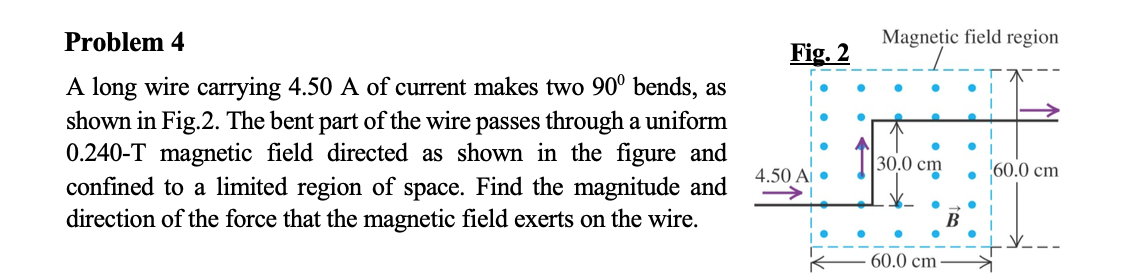Problem 4 Magnetic field region Fig. 2 A long wire carrying 4.50 A of current makes two 90° bends, as shown in Fig.2. The bent part of the wire passes through a uniform 0.240-T magnetic field directed as shown in the figure and confined to a limited region of space. Find the magnitude and direction of the force that the magnetic field exerts on the wire. . ||30.0 cm 60.0 cm 4.5 Y_ - 60.0 cm

• ### A long wire carrying 6.50 of current makes two bends, as shown in the figure ....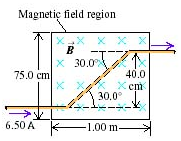A long wire carrying 6.50 of current makes two bends, as shown in the figure . The bent part of the wire passes through a uniform 0.280 magnetic field directed as shown in the figure and confined to a limited region of space. A) Find the magnitude of the force that the magnetic field exerts on the wire. B) Find the direction of the force that the magnetic field exerts on the wire. The answer to part A is 1.96...

• ### Magnetic field region Problem 5:* A long wire carrying 6.50 A of currents makes two bends, as sho...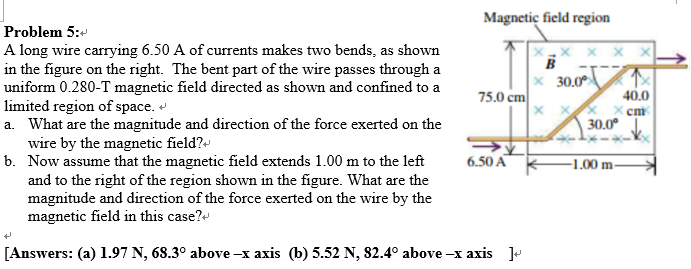Please No Handwriting! Thank you! :) Magnetic field region Problem 5:* A long wire carrying 6.50 A of currents makes two bends, as shown in the figure on the right. The bent part of the wire passes through a uniform 0.280-T magnetic field directed as shown and confined to a limited region of space. a. What are the magnitude and direction of the force exerted on the x30.0 40.0 x cm 75.0 cm 30.0° wire by the magnetic field?v Now...

• ### Need help with this question m one wire carrying 2.0 AOT current makes two 90° bends,...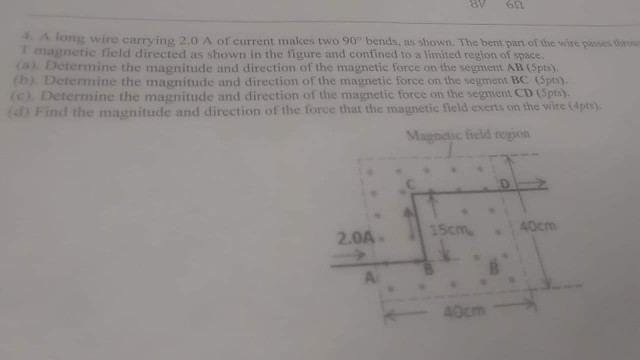Need help with this question m one wire carrying 2.0 AOT current makes two 90° bends, as shown. The bent part of the Web unette meld directed as shown in the figure and confined to a limited region of space Determine the magnitude and direction of the magnetic force on the seament AB (S) Determine the m onitude and direction of the mainetic force on the soment BC (p Determine the magnitude and direction of the mainetic force on the...

• ### Magnetic Fields and Forces

A long wire carrying 4.50 ofcurrent makes two 90 bends, as shown in the figure . The bentpart of the wire passes through a uniform 0.230 magnetic field directed as shown in the figure and confined to a limited region of space.A)Find the magnitude of the force that the magnetic field exerts on the wire.B)Find the direction of the force that the magnetic field exerts on the wire.

• ### NEED URGENT! Current Carrying ConductorA long wire carrying 6.50 of current makes two bends, as shown in the figure.The bent part of the wire passes through a uniform 0.280 magnetic field directed as shown in the figure andconfined toa limited region of space.A) Find the magnitude of the force that the magnetic fieldexerts on the wire.B) Find the direction of the force that the magnetic fieldexerts on the wire.

• ### A long wire carrying a 6.00A current reverses direction by means of two right-angle bends, as...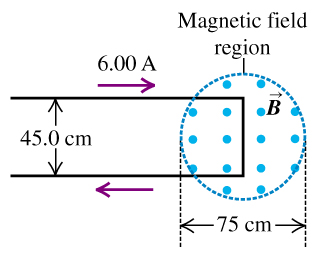A long wire carrying a 6.00A current reverses direction by means of two right-angle bends, as shown in the figure (Figure 1) . The part of the wire where the bend occurs is in a magnetic field of 0.666T confined to the circular region of diameter 75cm, as shown. A) Find the direction of the net force that the magnetic field exerts on this wire. B) Find the magnitude of the net force that the magnetic field exerts on this...

• ### Question   E:   Bent   Wire   in   Field Question E: Bent Wire in Field Phenomenon: A long wire...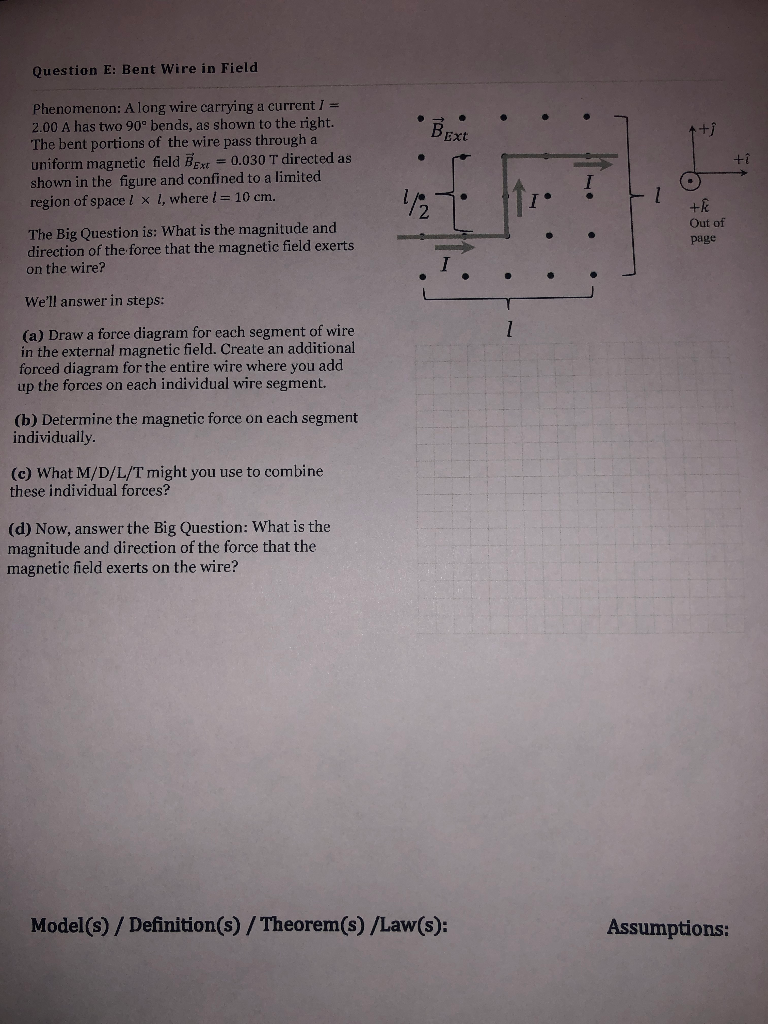Question   E:   Bent   Wire   in   Field Question E: Bent Wire in Field Phenomenon: A long wire carrying a current I 2.00 A has two 90° bends, as shown to the right. Ext The bent portions of the wire pass through a +1 uniform magnetic field B Ext-0.030 T directed as shown in the figure and confined to a limited /2 region of space 1 x 1, where l = 10 cm. Out of page The Big Question is: What is...